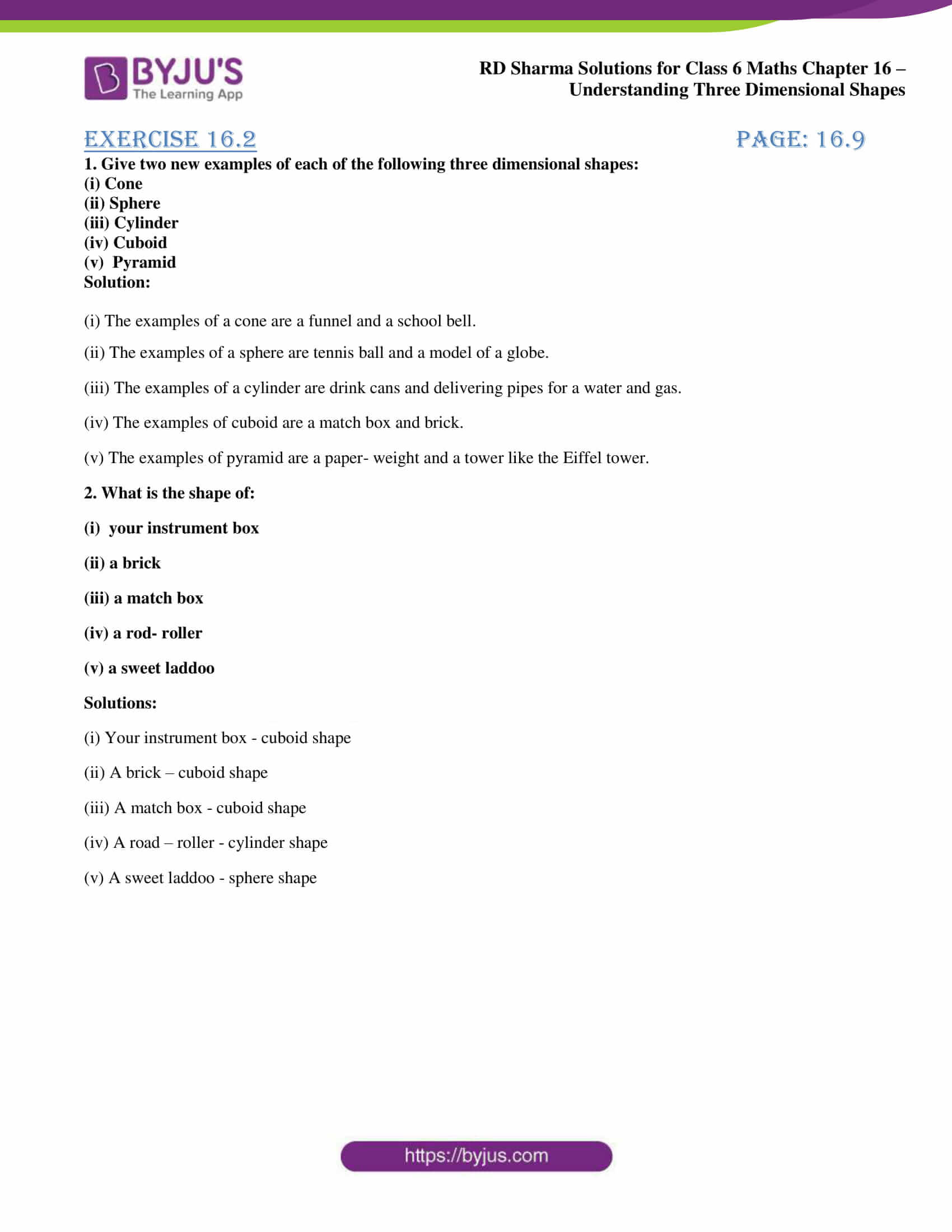# RD Sharma Solutions for Class 6 Maths Chapter 16: Understanding Three Dimensional Shapes Exercise 16.2

The students can score good marks in the exam by using solutions designed by teachers at BYJU’S. Exercise 16.2 of Chapter 16 mainly explains students about the major concepts like cylinders, right circular cone, sphere and pyramid based on the latest CBSE syllabus. The solutions are created in an interesting manner to make learning fun for the students. For further reference, students can make use of RD Sharma Solutions Class 6 Maths Chapter 16 Understanding Three Dimensional Shapes Exercise 16.2 PDF from the links which are provided here.

## RD Sharma Solutions for Class 6 Maths Chapter 16: Understanding Three Dimensional Shapes Exercise 16.2 Download PDF### Access answers to Maths RD Sharma Solutions for Class 6 Chapter 16: Understanding Three Dimensional Shapes Exercise 16.2

1. Give two new examples of each of the following three dimensional shapes:

(i) Cone

(ii) Sphere

(iii) Cylinder

(iv) Cuboid

(v)  Pyramid

Solution:

(i) The examples of a cone are a funnel and a school bell.

(ii) The examples of a sphere are tennis ball and a model of a globe.

(iii) The examples of a cylinder are drink cans and delivering pipes for a water and gas.

(iv) The examples of cuboid are a match box and brick.

(v) The examples of pyramid are a paper- weight and a tower like the Eiffel tower.

2. What is the shape of:

(ii) a brick

(iii) a match box

(iv) a rod- roller